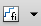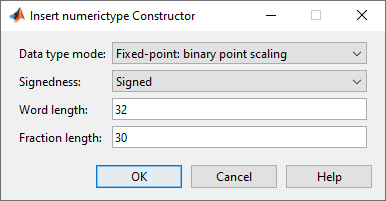## numerictype Object Construction

### numerictype Object Syntaxes

`numerictype` objects define the data type and scaling attributes of `fi` objects, as well as Simulink® signals and model parameters. You can create `numerictype` objects in Fixed-Point Designer™ software in one of two ways:

• You can use the `numerictype` constructor function to create a new object.

• You can use the `numerictype` constructor function to copy an existing `numerictype` object.

To get started, type

```T = numerictype ```

to create a default `numerictype` object.

```T = DataTypeMode: Fixed-point: binary point scaling Signedness: Signed WordLength: 16 FractionLength: 15 ```

To see all of the `numerictype` object syntaxes, refer to the `numerictype` constructor function reference page.

The following examples show different ways of constructing `numerictype` objects. For more examples of constructing `numerictype` objects, see the Examples on the `numerictype` constructor function reference page.

### Example: Construct a numerictype Object with Property Name and Property Value Pairs

When you create a `numerictype` object using property name and property value pairs, Fixed-Point Designer software first creates a default `numerictype` object, and then, for each property name you specify in the constructor, assigns the corresponding value.

This behavior differs from the behavior that occurs when you use a syntax such as `T = numerictype(s,w)`, where you only specify the property values in the constructor. Using such a syntax results in no default `numerictype` object being created, and the `numerictype` object receives only the assigned property values that are specified in the constructor.

The following example shows how the property name/property value syntax creates a slightly different `numerictype` object than the property values syntax, even when you specify the same property values in both constructors.

To demonstrate this difference, suppose you want to create an unsigned `numerictype` object with a word length of `32` bits.

First, create the `numerictype` object using property name/property value pairs.

`T1 = numerictype('Signed',0,'WordLength',32)`
```T1 = DataTypeMode: Fixed-point: binary point scaling Signedness: Unsigned WordLength: 32 FractionLength: 15```

The `numerictype` object `T1` has the same `DataTypeMode` and `FractionLength` as a default `numerictype` object, but the `WordLength` and `Signed` properties are overwritten with the values you specified.

Now, create another unsigned `32` bit `numerictype` object, but this time specify only property values in the constructor.

`T2 = numerictype(0,32)`
```T2 = DataTypeMode: Fixed-point: unspecified scaling Signedness: Unsigned WordLength: 32```

Unlike `T1`, `T2` only has the property values you specified. The `DataTypeMode` of `T2` is ```Fixed-Point: unspecified scaling```, so no fraction length is assigned.

`fi` objects cannot have unspecified `numerictype` properties. Thus, all unspecified `numerictype` object properties become specified at the time of `fi` object creation.

### Example: Copy a numerictype Object

To copy a `numerictype` object, simply use assignment as in the following example:

```T = numerictype; U = T; isequal(T,U)```
```ans = 1```

### Example: Build numerictype Object Constructors in a GUI

When you are working with files in MATLAB®, you can build your `numerictype` object constructors using the Insert numerictype Constructor dialog box. After specifying the properties of the `numerictype` object in the dialog box, you can insert the prepopulated `numerictype` object constructor at a specific location in your file.

For example, to create a signed `numerictype` object with binary-point scaling, a word length of 32 bits and a fraction length of 30 bits, perform the following steps:

1. On the Home tab, in the File section, click New > Script to open the MATLAB Editor

2. On the Editor tab, in the Edit section, clickin the Insert button group. Click the Insert numerictype... to open the Insert numerictype Constructor dialog box.

3. Use the edit boxes and drop-down menus to specify the following properties of the `numerictype` object:

• Data type mode = ```Fixed-point: binary point scaling```

• Signedness = `Signed`

• Word length = `32`

• Fraction length = `30`4. To insert the `numerictype` object constructor in your file, place your cursor at the desired location in the file, and click on the Insert numerictype Constructor dialog box. Clicking closes the Insert numerictype Constructor dialog box and automatically populates the `numerictype` object constructor in your file:##### SupportGet trial now